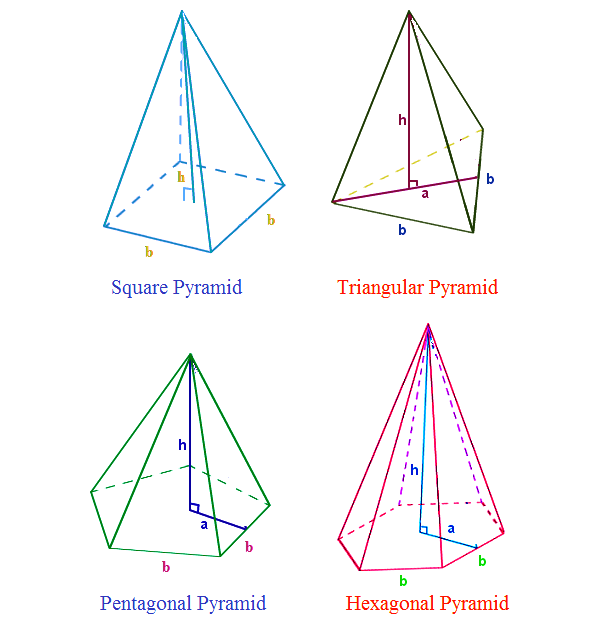# Volume of a Pyramid Formula

In geometry, a pyramid is a polyhedron formed by connecting a polygonal base and a point, called the apex. Each base edge and apex form a triangle called a lateral face. The volume of a pyramid is the measure of the number of units occupied by the pyramid.The Volume of a Pyramid Formula is given as,

$\large Volume\;of\;a\;square\;pyramid=\frac{1}{3}b^{2}h$

$\large Volume\;of\;a\;triangular\;pyramid=\frac{1}{6}abh$

$\large Volume\;of\;a\;pentagonal\;pyramid=\frac{5}{6}abh$

$\large Volume\;of\;a\;hexagonal\;pyramid=abh$

Where,
a – apothem length of the pyramid
b – base length of the pyramid
h – height of the pyramid

### Volume of a Pyramid Formula Solved Example

Example: A pyramid has a square base of side 4 cm and a height of 9 cm. Find its volume.

Solution:

Given,
b = 4 cm
h = 9 cm

$$\begin{array}{l}V=\frac{1}{3}b^{2}h\end{array}$$
$$\begin{array}{l}=\frac{1}{3}\times4^{2}\times9\end{array}$$
$$\begin{array}{l}=48\end{array}$$

So, the volume is

$$\begin{array}{l}48\,cm^{3}\end{array}$$
.

 More topics in Volume of a Pyramid Formula Volume of a Square Pyramid Formula